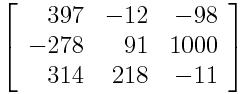# The power of parity

Puzzle: Give an elegant proof that the following matrix is invertible.Solution: The determinant of the matrix is odd, so the determinant is not zero, so the matrix is invertible.

Why is the determinant odd? The determinant is defined as a sum of products that pick an element from each row and each column. Some of the products are multiplied by -1, but that doesn’t matter for our purposes. Each product of three elements is even except for the product that takes the terms along the diagonal, which are all odd. The sum of an odd number and several even numbers is odd.

In full detail, the determinate is

397×91×(-11) + (-12)×1000×314 + (-98)×(-278)×218 – (-98)×91×314 – (-12)×(-278)×(-11) – 397×218×1000

One odd number plus five even numbers is an odd number.

I saw this example in a course by Jeff Vaaler years ago.

For more examples of problems trivialized by parity arguments, see Saved by symmetry.

Related post: Odd numbers in odd bases

## 3 thoughts on “The power of parity”

1. Your proof first takes the determinant and then reduces mod 2. It may be easier to go the other way:

Lemma: If an integer matrix is invertible when reduced mod p, it is invertible. Proof: if not, some nontrivial rational combination of the columns is zero; hence some nontrivial integer combination is; hence, some integer combination whose coefficients have no common factor. Now reduce this mod p. []

And now just notice that the matrix becomes the identity on reducing mod 2.

(Question: the proof of the lemma is very easy, but can it be made *absolutely blindingly obvious*?)

2. det (A (mod n)) \congruent det(A) (mod n)

3. Very interesting comment of Mc Caughan above !